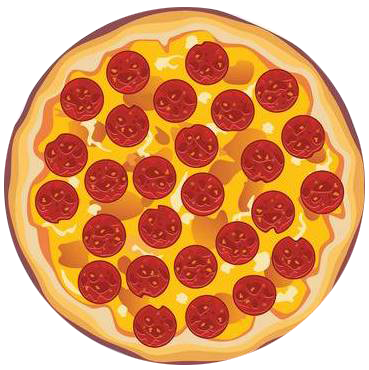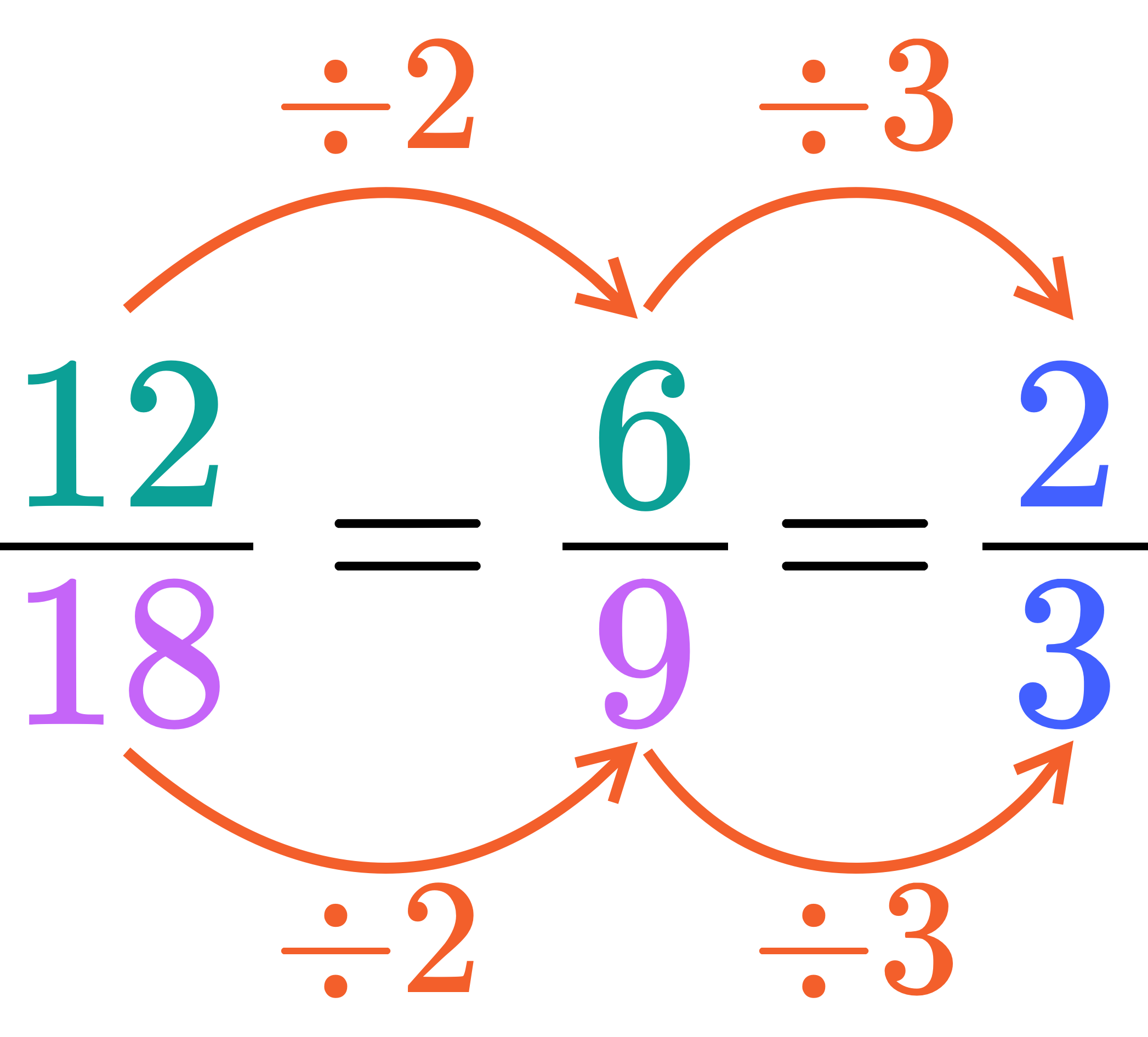IntroductionIntroduction# Simplifying Fractions

To help us better understand fractions, we like to imagine them as pizzas or legos.

Pick whichever you vibe with more:

INTRO
A simplified (or reduced) fraction is a fraction written in simplest form, meaning the only number that evenly divides into both the top and bottom is .
In pizza terms, when we simplify a fraction, we want to determine the fewest number of equal slices we can cut a portion of pizza into without changing the amount of pizza.Simplifying a fraction does not change its value, meaning the simplified fraction is equivalent to the original fraction. Check out equivalent fractions below by dragging the blue line:
Check out our
Calculator
or explore our
Lesson
and
Practice

CALCULATOR

KEY STEPS

## How to Simplify Fractions

### Step 1. Divide the top and bottom by the same number.

The number should be a common factor, or a number that divides evenly into both the top and bottom.

### Step 2. Repeat Step 1 until the only common factor left is .

Keep dividing the top and bottom by a common factor until the only one left is .
We can also just find the greatest common factor, and divide both the top and bottom by it. This guarantees us the most simplified version of the fraction.
LESSON
Simplifying Fractions

## Simplifying Fractions

Simplifying (or reducing) a fraction means making the top and bottom as small as possible without changing the value of the fraction.

We can do this by dividing the top and bottom of the fraction by common factors until the only common factor left is .### What is a common factor?

A common factor is a number that evenly fits into two or more given numbers.
And remember, factors always come in pairs consisting of the factor and the number of times it fits evenly into the given number.
 Factors of : Factors of : The common factors of and are and .
Now that we know our basics, let’s check out some examples of how to simplify fractions using common factors!
PRACTICE
Simplifying Fractions

## Practice: Simplifying Fractions

Question 1 of 3: Simplify .

### Step 1. Divide the top and bottom by the same number.

The number should be a common factor, or a number that divides evenly into both the top and bottom.

CONCLUSION
Incredible job, look at you go! Thanks for checking out this lesson ☺️🙏. Where to next?Leave Feedback